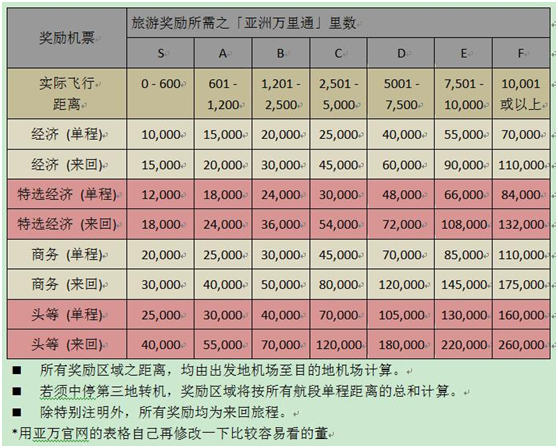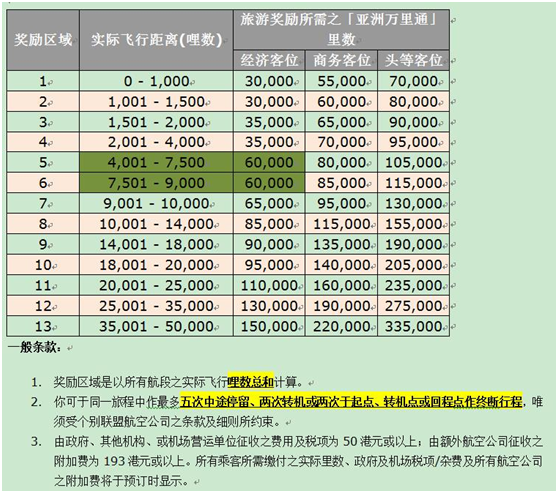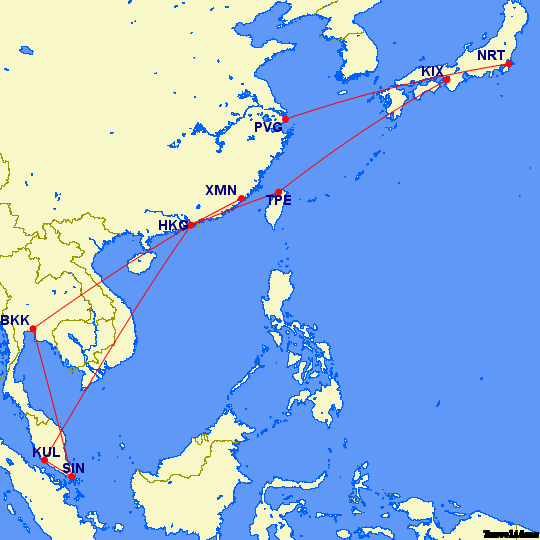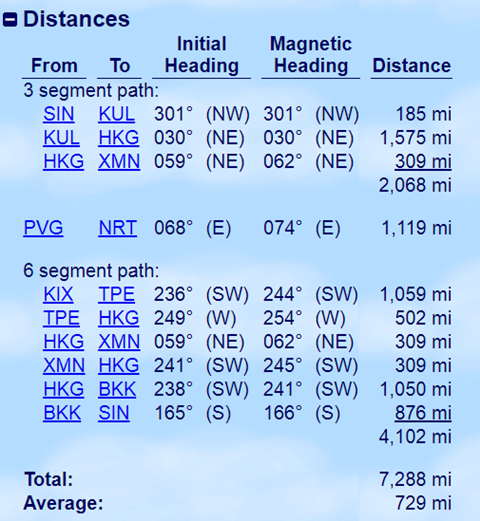# Asia Miles亚洲万里通 60K Miles 玩遍新马泰日台港

Asia Miles亚洲万里通 60K Miles 玩遍新马泰日台港

「亚洲万里通」奖励换算表「寰宇一家」

「寰宇一家」奖励换算表###重点来了###

A=>B

A=>B(转机/停留)=>C

A=>B(停留)=>C(停留)=>B(转机)=>A

A=>B(转机)=>C(停留)=>D(停留)=>B(转机)=>A

A=>B(开口) C=>A

A=>B(转机)=>C (目的地开口)D=>E(停留)=>B(转机)=>A

A=>B(转机)=>C(停留)=>D (目的地开口)E=>F(停留)=>B(转机)=>A

PVG=>NRT(转机)=>CTS(停留)=>NGO(目的地开口) KIX=>TPE(停留)=>
HKG(转机)=>PVG(30K经济 50K商务)

A=>B(转机)=>C(停留)=>F(停留)=>B(转机)=>G (出发地开口)

PVG=>NRT(转机)=>CTS(停留)=>KIX(停留)=>HKG(转机)=>XMN(开口)

30K经济 50K商务

30K有没有很超值

SIN=>KUL(停留)=>HKG(转机)=>XMN(开口)PVG=>NRT (开口)KIX=>TPE(停留)=>HKG(转机)=>XMN(停留)=>HKG(停留)=>BKK(停留)=>SIN